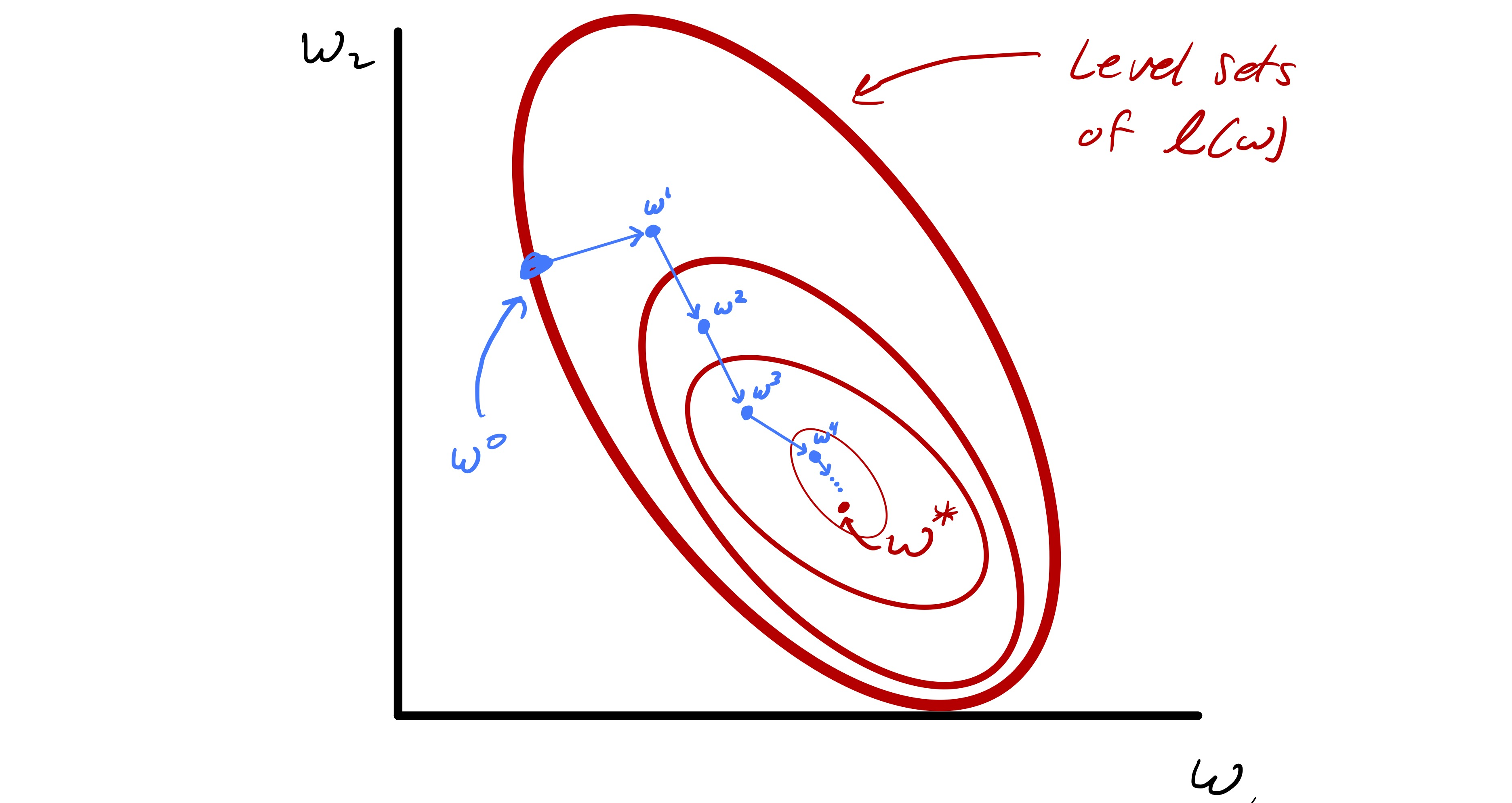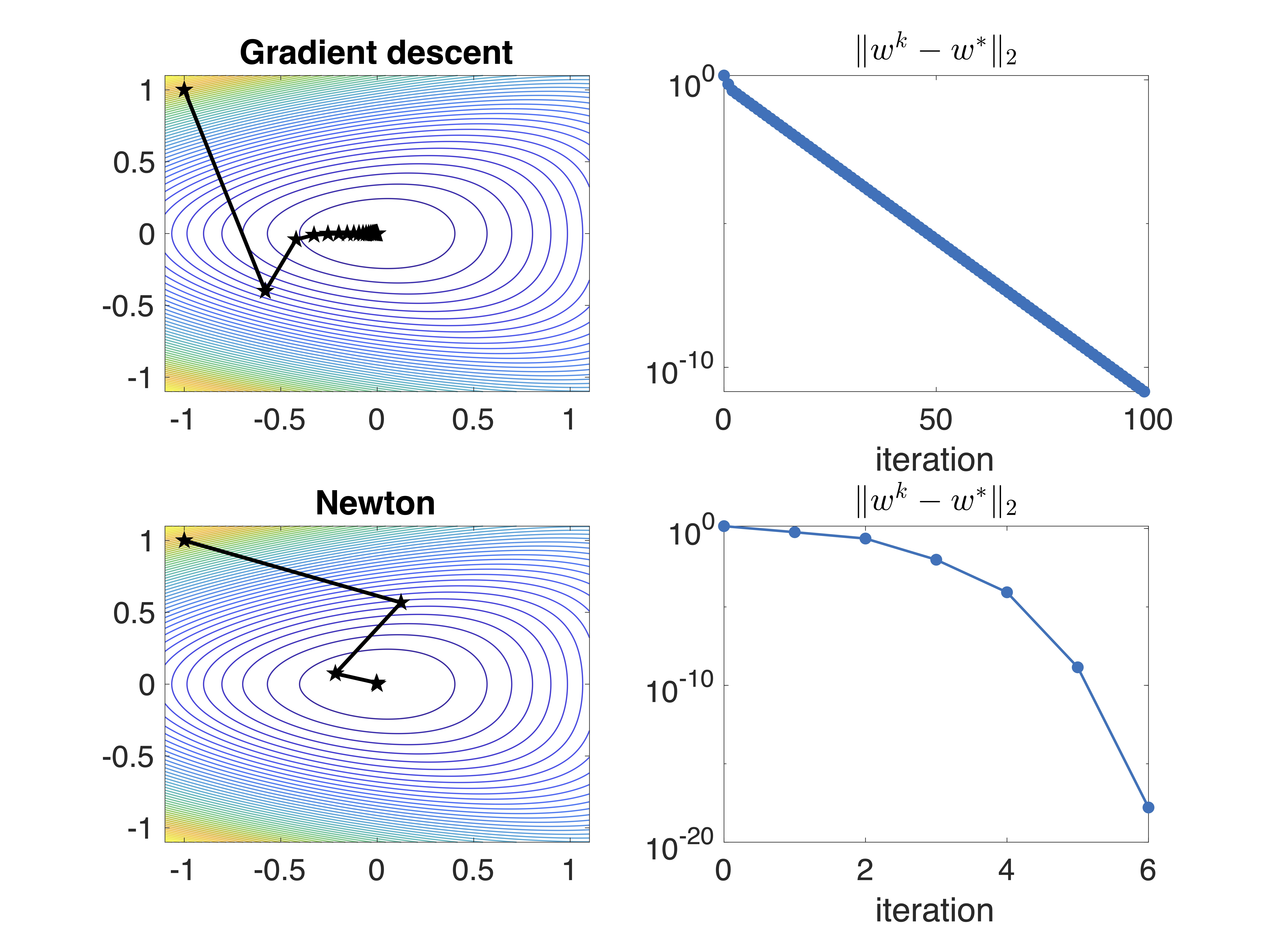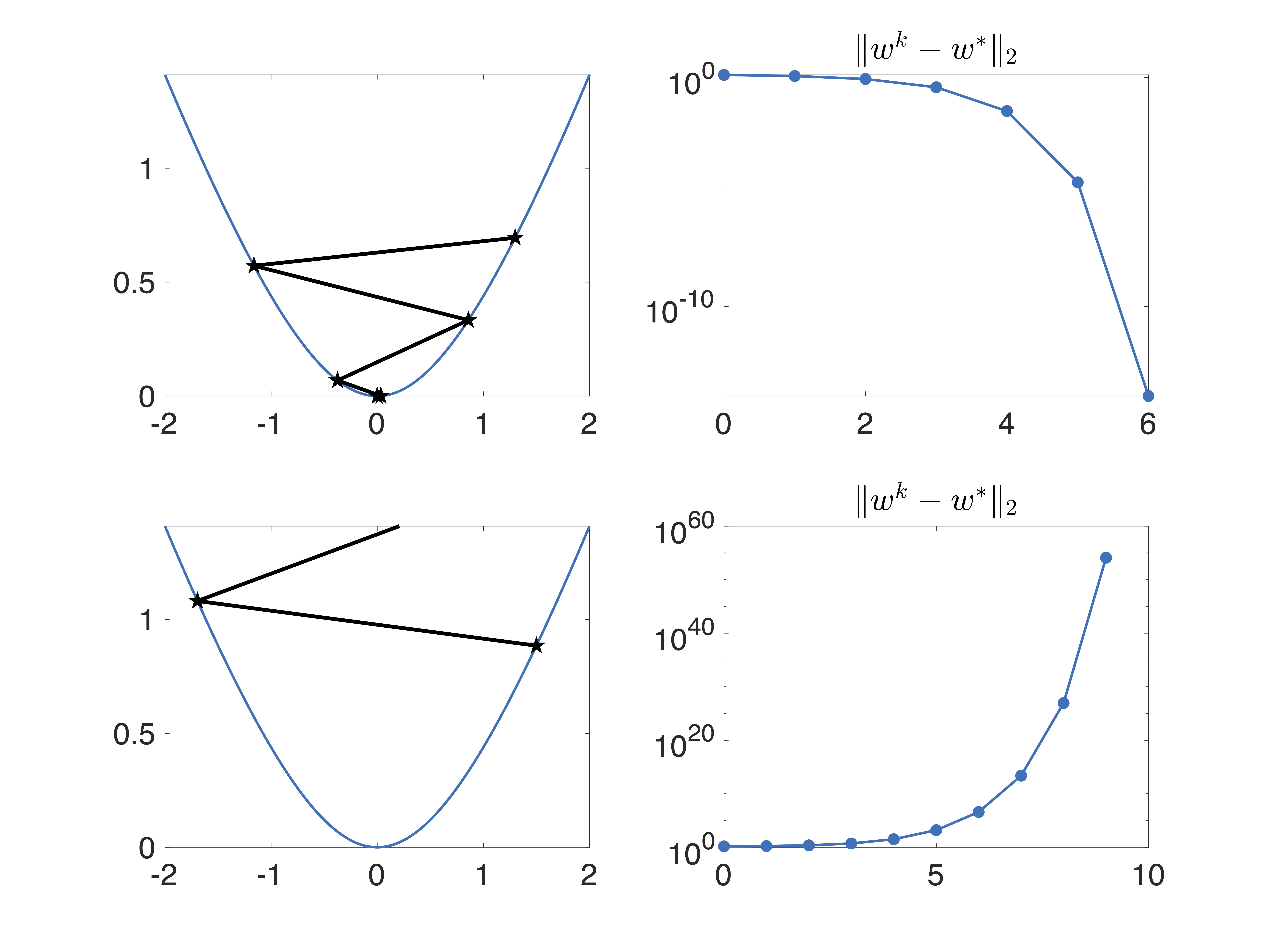Fall 2021

In the previous lecture on Logistic Regression we wrote down expressions for the parameters in our model as solutions to optimization problems that do not have closed form solutions. Specifically, given data $$\{(x_i,y_i)\}_ {i=1}^n$$ with $$x_i\in\mathbb{R}^d$$ and $$y_i\in\{+1,-1\}$$ we saw that $\hat{w}_{\text{MLE}} = \mathop{\mathrm{arg\,min}}_{w\in\mathbb{R}^d,b\in\mathbb{R}}\; \sum_{i=1}^n \log(1+e^{-y_i(w^Tx_i+b)})$(1)
and $\hat{w}_{\text{MAP}} = \mathop{\mathrm{arg\,min}}_{w\in\mathbb{R}^d,b\in\mathbb{R}}\; \sum_{i=1}^n \log(1+e^{-y_i(w^Tx_i+b)}) + \lambda w^Tw.$(2)

These notes will discuss general strategies to solve these problems and, therefore, we abstract our problem to $\min_{w} \ell(w),$(3) where $$\ell\colon \mathbb{R}^d \rightarrow \mathbb{R}.$$ In other words, we would like to find the vector $$w$$ that makes $$\ell(w)$$ as small as possible. This is a very general problem that arises in a broad range of applications and often the best way to approach it depends on properties of $$\ell.$$ While we will discuss some basic algorithmic strategies here, in practice it is important to use well developed software—there are a lot of little details that go into practically solving an optimization problem and we will not cover them all here. Numerical optimization is both a very well studied area and one that is continually evolving.

Before diving into the mathematical and algorithmic details we will make some assumptions about our problem to simplify the discussion. Specifically, we will assume that:

1. $$\ell$$ is convex. This allows us to assert that any local minimum we find is also a global minimum,1 and helps simplify our discussion of Newton’s method.
2. $$\ell$$ is at least thrice continuously differentiable. We are going to extensively use Taylor approximations and this assumption simplifies the discussion greatly.
3. There are no constraints placed on $$w.$$ It is common to consider the problem $$\min_{w\in\mathcal{C}}\ell(w)$$ where $$\mathcal{C}$$ represents some constraints on $$w$$ (e.g., $$w$$ is entrywise non-negative). Adding constraints is a level of complexity we will not address here—it is best left to a course that focuses on numerical optimization.

What is a (local) minimizer

The first question we might ask is what it actually means to solve eq. 3. We call $$w^*$$ a local minimizer of $$\ell$$ if:

There is some $$\epsilon > 0$$ such that for $$\{w \,\vert\, \|w-w^*\|_ 2 < \epsilon\}$$ we have that $$\ell(w^*) \leq \ell(w ).$$

In other words, there is some small radius around $$w^*$$ where $$w^*$$ makes $$\ell$$ as small as possible. It turns out that our earlier assumption that $$\ell$$ is convex implies that if we find such a $$w^*$$ we can immediately assert that $$\ell(w^*) \leq \ell(w)$$ for all $$w\in\mathbb{R}^d.$$ Note that none of the inequalities involving $$\ell$$ are strict, we can also define a strict local minimizer by forcing $$\ell(w^*) < \ell(w).$$2 Notably, some convex functions have no strict local minimizers, e.g., the constant function $$\ell(w) = 1$$ is convex, and some convex functions have no finite local minimizers, e.g., for any non-zero vector $$c\in\mathbb{R}^d$$ $$c^Tw$$ is convex but can be made arbitrarily small by letting $$\|w\|_ 2\rightarrow\infty$$ appropriately.

Mathematically, we often talk about (first and second order) necessary and sufficient conditions for a point to be a local minimizer. We will omit many of the details here, but a key necessary condition is that the gradient of $$\ell$$ is zero at $$w^*,$$ i.e., $$\nabla\ell(w^*) = 0.$$ If not, we can actually show there is a direction we can move in that further decreases $$\ell.$$ Assuming the gradient at $$w^*$$ is zero, a sufficient condition for the point to be a strict local minimizer is for the Hessian, i.e., $$\nabla^2\ell(w^*)$$ to be positive definite.

Taylor expansions

While we made some assumptions on $$\ell,$$ they do not actually tell us much about the function—it could behave in all sorts of ways. Moreover, as motivated by the logistic regression example it may not be so easy to work with the function globally. Therefore, we will often leverage local information about the function $$\ell.$$ We accomplish this through the use of first and second order Taylor expansions.3

Recall that the first order Taylor expansion of $$\ell$$ centered at $$w$$ can be written as $\ell(w+p) \approx \ell(w) + g(w)^Tp,$(4) where $$g(w)$$ is the gradient of $$\ell$$ evaluated at $$w,$$ i.e., $$(g(w))_ j = \frac{\partial \ell}{\partial w_j}(w)$$ for $$j=1,\ldots,d.$$ Similarly, the second order Taylor expansion of $$\ell$$ centered at $$w$$ can be written as $\ell(w+p) \approx \ell(w) + g(w)^Tp + \frac{1}{2}p^T H(w)p,$(5) where $$H(w)$$ is the Hessian of $$\ell$$ evaluated at $$w,$$ i.e., $$[H(w)]_ {i,j} = \frac{\partial^2 \ell}{\partial w_i\partial w_j}(w)$$ for $$i,j = 1,\ldots,d.$$ These correspond to linear and quadratic approximations of $$\ell$$ as illustrated in fig. 1. In general, these approximations are reasonably valid if $$p$$ is small (concretely, eq. 4 has error $$\mathcal{O}(\|p\|_ 2^2)$$ and eq. 5 has error $$\mathcal{O}(\|p\|_ 2^3)$$).

Search direction methods

The basic approach we will take to solving eq. 3 here fall into the broad category of search direction methods. The core idea is that given a starting point $$w^0$$ we construct a sequence of iterates $$w^1,w^2,\ldots$$ with the goal that $$w^k\rightarrow w^*$$ as $$k\rightarrow \infty.$$ In a search direction method we will think of constructing $$w^{k+1}$$ from $$w^{k}$$ by writing it as $$w^{k+1} = w^{k} + s$$ for some “step” $$s.$$ Concretely, this means our methods will have the following generic format:

Input: initial guess $$w^0$$

k = 0;

While not converged:

1. Pick a step $$s$$
2. $$w^{k+1} = w^{k}+s$$ and $$k = k + 1$$
3. Check for convergence; if converged set $$\hat{w} = w^{k}$$

Return: $$\hat{w}$$

This process is schematically outlined in fig. 2.Figure 2: A search direction method applied to optimize a function of two variables.

There are two clearly ambiguous steps in the above algorithm: how do we pick $$s$$ and how do we determine when we have converged. We will spend most of our time addressing the former and then briefly touch on the latter—robustly determining convergence is one of the little details that a good optimization package should do well.

The core idea behind gradient descent can be summed up as follows: given we are currently at $$w^k,$$ determine the direction in which the function decreases the fastest (at this point) and take a step in that direction. Mathematically, this can be achieved by considering the linear approximation to $$\ell$$ at $$w^k$$ provided by the Taylor series. Specifically, if we consider the linear function $\ell(w^k) + g(w^k)^Ts$ then the fastest direction to descend is simply $$s \propto -g(w^k).$$ However, the linear approximation does not tell us how far to go (it runs off to minus infinity). Therefore, what we actually do in gradient descent is set $$s$$ as $s = -\alpha g(w^k)$ for some step size $$\alpha > 0.$$ The linear approximation is only good locally, so it is not actually clear that this choice even decreases $$\ell$$ if $$\alpha$$ is chosen poorly. However, assuming the gradient is non-zero we can show that there is always some small enough $$\alpha$$ such that $$\ell(w^k-\alpha g(w^k)) < \ell(w^k).$$ In particular, we have that $\ell(w^k-\alpha g(w^k)) = \ell(w^k) - \alpha g(w^k)^Tg(w^k) + \mathcal{O}(\alpha^2).$ Since $$g(w^k)^Tg(w^k) > 0$$ and $$\alpha^2 \rightarrow 0$$ faster than $$\alpha$$ as $$\alpha\rightarrow 0$$ we conclude that for some sufficiently small $$\alpha > 0$$ we have that $$\ell(w^k-\alpha g(w^k)) < \ell(w^k).$$

In classical optimization, $$\alpha$$ is often referred to as the step size (in this case $$g(w^{k})$$ is the search direction). However, in machine learning $$\alpha$$ is typically referred to as the learning rate. In numerical optimization there are good strategies for picking $$\alpha$$ in an adaptive manner at each step. However, they can be more expensive then a fixed strategy for setting $$\alpha.$$ The catch is that setting $$\alpha$$ too small can lead to slow convergence and setting $$\alpha$$ too large can actually lead to divergence—see fig. 3. A safe choice that guarantees convergence is to set $$\alpha = c/k$$ at iteration $$k$$ for any constant $$c>0.$$

One related strategy is to set the step size per entry of the gradient; though this is actually best thought of as modifying the step direction on a per entry basis. Adagrad (technically, diagonal Adagrad) accomplishes this by keeping a running average of the squared gradient with respect to each optimization variable. It then sets a small learning rate for variables with large gradients and a large learning rate for features with small gradients. This can be important if the entries of $$w$$ are attached to features (e.g., in logistic regression we can associate each entry of $$w$$ with a feature) that vary in scale or frequency.

Input: $$\ell,$$ $$\nabla\ell,$$ parameter $$\epsilon > 0,$$ and initial learning rate $$\alpha.$$

Set $$w^0_j=0$$ and $$z_j=0$$ for $$j=1,\ldots,d.$$ k = 0;

While not converged:

1. Compute entries of the gradient $$g_j = \frac{\partial \ell}{\partial w_j}(w^k)$$
2. $$z_j = z_j + g_j^2$$ for $$j=1,\ldots,d.$$
3. $$w^{k+1}_ j = w^{k}_ j - \alpha\frac{g_j}{\sqrt{z_j+\epsilon}}$$ for $$j=1,\ldots,d.$$
4. $$k = k+1$$
5. Check for convergence; if converged set $$\hat{w} = w^{k}$$

Return: $$\hat{w}$$

Newton’s method

In gradient descent we used first order information about $$\ell$$ at $$w^k$$ to determine which direction to move. A natural follow-up is then to use second order information—this leads to Newton’s method. Specifically, we now chose a step by explicitly minimizing the quadratic approximation to $$\ell$$ at $$w^k.$$ Recall that because $$\ell$$ is convex $$H(w)$$ is positive semi-definite for all $$w,$$ so this is a sensible thing to attempt.4 In fact, Newton’s method has very good properties in the neighborhood of a strict local minimizer and once close enough to a solution it converges rapidly.

To derive the Newton step consider the quadratic approximation $\ell(w^k+s) \approx \ell(w^k) + g(w^k)^Ts + \frac{1}{2}s^T H(w^k)s.$ Since this approximation is a convex quadratic we can pick $$s$$ such that $$w^{k+1}$$ is a local minimizer. To accomplish this we explicitly solve $\min_s \ell(w^k) + g(w^k)^Ts + \frac{1}{2}s^T H(w^k)s$ by differentiating and setting the gradient equal to zero. For simplicity, lets assume for the moment that $$H(w^k)$$ is positive definite.5 Since the gradient of our quadratic approximation is $$g(w^k)+H(w^k)s$$ this implies that $$s$$ solves the linear system $H(w^k)s = -g(w^k).$ In practice there are a many approaches that can be used to solve this system, but it is important to note that relative to gradient descent Newton’s method is more expensive as we have to compute the Hessian and solve a $$d\times d$$ linear system.

A simple example

While we will discuss the pros and cons of Newton’s method in the next section, there is a simple example that clearly illustrates how incorporating second order information can help. Pretend the function $$\ell$$ was actually a strictly convex quadratic, i.e., $\ell(w) = \frac{1}{2}w^TAw + b^Tw + c$ where $$A$$ is a positive definite matrix, $$b$$ is an arbitrary vector, and $$c$$ is some number. In this case, Newton converges in one step (since the strict global minimizer $$w^*$$ of $$\ell$$ is the unique solution to $$Aw = b$$).

Meanwhile, gradient descent yields the sequence of iterates $w^{k} = (I - \alpha A)w^{k-1} - \alpha b.$ Using the fact that $$w^*= (I-\alpha A)w^*- \alpha b$$ we can see that \begin{align} \|w^{k} - w^*\| &\leq \|I - \alpha A\|_ 2 \|w^{k-1} - w^*\|_ 2 \\ &\leq \|I - \alpha A\|_ 2^k \|w^{0} - w^*\|_ 2. \end{align} Therefore, as long as $$\alpha$$ is small enough such that all the eigenvalues of $$I - \alpha A$$ are in $$(-1,1)$$ the iteration will converge—albeit slowly if we have eigenvalues close to $$\pm 1$$. More generally, fig. 4 shows an example where we see the accelerated convergence of Newton’s method as we approach the local minimizer.Figure 4: Comparison of gradient descent and Newton’s method; in this case the function and starting point are favorable to Newton’s method.

Potential issues

While Newton’s method converges very quickly once $$w^k$$ is sufficiently close to $$w^*,$$ that does not mean it always converges (see fig. 5) In fact, you can set up examples where it diverges or enters a limit cycle. This typically means the steps are taking you far from where the quadratic approximation is valid. Practically, a fix for this is to introduce a step size $$\alpha > 0$$ and formally set $$s = -\alpha [H(w^k)]^{-1}g(w^k).$$ We typically start with $$\alpha = 1$$ since that is the proper step to take if the quadratic is a good approximation. However, if this step seems poor (e.g., $$\ell(w^{k}+ s) > \ell(w^{k})$$) then we can decrease $$\alpha$$ at that iteration.Figure 5: Example where Newton’s method converges or diverges depending on the starting point.

Another issue that we sidestepped is that when $$\ell$$ is convex but not strictly convex we can only assume that $$H(w^k)$$ is positive semi-definite. In principle this means that $$H(w^k)s = -g(w^k)$$ may not have a solution. Or, if $$H(w^k)$$ has a zero eigenvalue then we always have infinite solutions. A “quick fix” is to simply solve $$(H(w^k) + \epsilon I)s = -g(w^k)$$ instead for some small parameter $$\epsilon.$$ This lightly regularizes the quadratic approximation to $$\ell$$ at $$w^k$$ and ensures it has a strict global minimizer.6

Checking for convergence

In the above meta algorithm we simply defined the sequence of iterates $$w^0,w^1,\ldots .$$ However, even in exact arithmetic one of the iterates will likely never be a local minimizer $$w^*$$—if that somehow happens we just got lucky or had a very special function. Rather, all we hope for is $$w^k\rightarrow w^*$$ as $$k\rightarrow \infty.$$ Therefore, we need rules to determine when to stop. Doing so can be delicate in practice, we don’t know how the function $$\ell$$ looks like. Some common factors that go into assessing convergence include:

1. Relative change in the iterates, i.e., $$\frac{\|w^{k+1}-w^k\|_ 2}{\|w^k\|_ 2} < \delta_1$$ for some small $$\delta_! > 0.$$
2. A reasonably small gradient, i.e., $$\|g(w^k)\|_ 2 < \delta_2$$ for some small $$\delta_2 > 0.$$

Both strategies have their failure modes, so in practice composite conditions are often used to ensure convergence has been reached.

Best practices and extensions

While we are covering a reasonably narrow slice of numerical optimization here (see, e.g., CS 4220 for a more thorough treatment), there are a few miscellaneous points worth stressing. Moreover, as illustrated in the class demo

While Newton’s method has very good local convergence properties, it is considerably more expensive than gradient descent in practice. We have to both compute the Hessian matrix and solve a $$d\times d$$ linear system at each step. Therefore, a lot of time has been devoted to the construction of so-called quasi-Newton methods. Generically, these methods compute a step $$s$$ by solving the linear system $M_ks = -g(w^k)$ where $$M_k\approx H(w^k)$$ is some sort of approximation to the Hessian that is easier to compute and yields a linear system that is easier to solve.

For example, We could let $$M_k$$ be the diagonal of the Hessian, i.e., $$[M_k]_ {i,i} = [H(w^k)]_ {i,i}$$ and $$[M_k]_ {i,j} = 0$$ if $$i\neq j.$$ Now we only have to compute $$d$$ entries of the Hessian and solving the associated linear system only requires computing $$s_i = -[g(w^k)]_ i / [H(w^k)]_ {i,i}$$ for $$i=1,\ldots,d.$$ In fact, Adagrad can be viewed through this lens where $$M_k$$ is a diagonal matrix with $$[M(w^k)]_ {i,i} = \frac{\alpha}{\sqrt{z_i+\epsilon}}.$$ These are only some simple examples, there are many more.

Sometimes we can mix methods, for example we could start with gradient descent to try and get near a local minimizer and then switch to Newton’s method to rapidly converge. This is cheaper than the idea above where we introduced a step size since we omit even computing the Hessian till we think it may help us. There are ways to formalize these sort of ideas that we will not cover here.

1. All the methods we discuss here are also applicable to nonconvex problems. However, in that setting we only look for local solutions and recognize that when/if we find one it may not be the global minimizer of our function.↩︎

2. If we further assume our function is strictly convex then we can say similar things about strict local and global minimizers.↩︎

3. Here, we omit an explicit discussion of error terms, but they are sometimes necessary in the analysis of optimization methods.↩︎

4. This can also be a sensible thing to do when $$\ell$$ is not convex. However, some modifications have to be made since the best quadratic approximation to $$\ell$$ may not be a convex quadratic and, therefore, may not have a finite local minimizer. This often takes the form of a modification of the Hessian to get a convex quadratic we can minimize.↩︎

6. Formally speaking this is not necessary. If $$g(w^k)$$ is in the range of $$H(w^k)$$ and $$H(w^k)$$ has a zero eigenvalue (so there are infinite solutions), we can simply pick the solution of minimal norm. This moves us to the local minimizer of the quadratic approximation closest to $$w^k.$$ Similarly, if $$g(w^k)$$ is not in the range of $$H(w^k)$$ then we can just take a gradient step. In fact, if $$g(w^k)$$ is not in the range of $$H(w^k)$$ it implies that the quadratic has no finite minimizer. So, a gradient step is a sensible thing to do. Moreover, if $$\ell$$ has a strict local minimizer then $$H(w)$$ is guaranteed to be positive definite once $$w$$ is close enough to the minimizer and this all becomes a moot point.↩︎